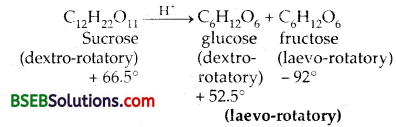# Bihar Board Class 12 Chemistry Solutions Chapter 4 Chemical Kinetics

Bihar Board Class 12 Chemistry Solutions Chapter 4 Chemical Kinetics Textbook Questions and Answers, Additional Important Questions, Notes.

## BSEB Bihar Board Class 12 Chemistry Solutions Chapter 4 Chemical Kinetics

### Bihar Board Class 12 Chemistry Chemical Kinetics Text Book Questions and AnswersQuestion 1.
For the reaction R → P, the concentration of a reactant changes from 0.03 M to 0.02M in 25 minutes. Calculate the average rate of reaction using units of time both in minutes and seconds.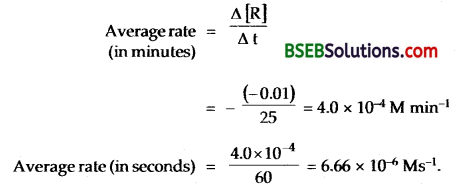Question 2.
In a reaction 2A → Products, the concentration of A decreases from 0.5 mol L-1 to 0.4 mol L-1 in 10 minutes. Calculate the rate during the reaction.
Rate of reaction = rate of disappearance of A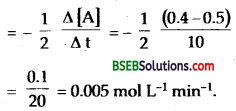Question 3.
For a reaction, A + B → Product, the rate law is given by r = k [A]1/2 [B]2 what is the order of the reaction?
Order of a reaction is the sum of the powers to which the concentrations of the reactants have to be raised in order to specify the rate of the reaction
r = k [A]1/2 [B]2
Order of the reaction = $$\frac { 1 }{ 2 }$$ + 2 = 2$$\frac { 1 }{ 2 }$$Question 4.
The conversion of molecules X to Y follows second order kinetics. If concentration of x is increased to three times, how will it affect the rate of formation of Y?
X → Y
Rate = k[X]2 … (i)
If cone of x is increased to 3 times
[X’] = [3X]
new rate will be = k [3X]2
= k.9 [X]2 = 9.k [X]2 … (ii)
From (i) and (ii) new rate will be 9 times.

Question 5.
A first order reaction has a rate constant 1.15 × 10-3 s-1, How long will 5g of this reactant take to reduce to 3g?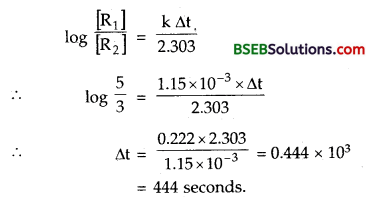Question 6.
Time required to decompose SO2 Cl2 to half of its inital amount is 60 minutes. If the decomposition is a first order reaction, calculate the rate constant of the reaction.
t1/2 for SO2 Cl2 = 60 min = 60 × 60 sec
Since it is in first order reaction
∴ k = $$\frac{0.693}{\mathrm{t}_{1 / 2}}$$ = $$\frac{0.693}{60 \times 60}$$
∴ rate constant of the reaction = 1.925 × 10-4 s-1.

Question 7.
What will be the effect of temperature on rate constant?
Most of the chemical reactions are accelerated by increase in temperature, whether the reactions are endothermic or exothermic. In a mixture of KMnO4and H2C2O4, potassium permangnate (KMnO4) gets decolourised faster at higher temperature than at a lower temperature. It has been found that for a rise of 10° in temp., the rate constant of of a reaction is nearly doubled. The temperature dependence of the rate of a chemical reaction can be accurately explained by Arrhenius equation
k = Ae-Ea/RT
Where A is the Arrhenius or frequency factor. It is also called PRE-EXPONENTIAL factor. It is a constant specific to a reaction.
R = Gas constant; Ea is the activation energy measured in joules mole.Question 8.
The rate of the chemical reaction doubles for an increase of’ 10 K in absolute temperature from 298 K. Calculate Ea.
T1 = 298 K
T2 = 298 + 10 = 308 K
R = 8.314 JK-1 mol-1
If initial rate constant is k1, then for 10° rise in temp.
final rate constant = 2k,
∴ putting these values in Arrhenius equation.Question 9.
The activation energy for the reaction 2HI (g) → H2 + I2, (g) is 2.09.5 kj mol-1 at 581 K. Calculate the fraction of the molecules of reactants having energy equal to or greater than activation energy.
Fraction of molecules with energy equal to or greater than activation energy, x = e-Ea/RT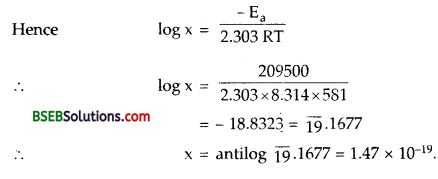### Bihar Board Class 12 Chemistry Chemical Kinetics Text Book Questions and Answers

Question 1.
From the rate expression for the following reactions determine their order of reaction and the dimensions of the rate constants.
(i) 3NO(g) → N2(g); Rate = k[NO]2
(ii) H2O2(aq) + 3I(aq) + 2H+ → 2H2O(I)3 ; Rate = k[H2O2] [I]
(iii)CH3CHO(g) → CH4(g) + CO(g) ; Rate = k[CH3CHO]3/2
(iv) C2H5Cl(g) → C2H4(g) + HCl(g); Rate = k[C2H5Cl]Question 2.
For the reaction 2A + B → A2B the rate = k[A][B]2 with k = 2.0 x 10-6 mol-2 L2 S-1. Calculate the initial rate of the reaction when [A] = 0.1 Mol L-1, [B] = 0.2 Mol L. Calculate the rate of the reaction after [A] is reduced to 0.6 mol-1.
(i) Intial rate = k[A][B]2
= (2.0 ✕ 10-6 mol-2 L2 s-1) (0.1 mol L-1) (0.2 mol L-1)2
= 8.0 ✕ 10-9 mol L-1 s-1

(ii) When [A] is reduced from 0.1 mol L-1 to 0.06 mol L-1
i.e. (0.1 – 0.06) = 0.04 mol L-1 has reacted, B reacted
$$\frac { 1 }{ 2 }$$ x 0.04 molL-1
= 0.02 mol L-1
Hence new [B] = (0.2 – 0.02) = 0.18 mol L-1
Now, rate = k[A][B]2
= (2.0 × 10-6 mol-2 L2 s-1) × (0.06 mol L-1) (0.18 mol L-1)2
= 3.89 × 10-9 mol L-1 s-1

Question 3.
The decomposition of NH3 on platinum surface is zero order reaction. What are the rates of production of N2 and H2 if k = 2.50 × 10-4 mol L S-1 ?
2NH3 (g) ⇌ N2(g) + 3H2(g)
rate = – $$\frac { 1 }{ 2 }$$.$$\frac{\Delta\left(\mathrm{NH}_{3}\right)}{\Delta \mathrm{t}}$$ = $$\frac{\Delta\left(\mathrm{N}_{2}\right)}{\Delta \mathrm{t}}$$ = $$\frac { 1 }{ 3 }$$ $$\frac{\Delta\left(\mathrm{H}_{2}\right)}{\Delta \mathrm{t}}$$
rate = k as it is a zero order reaction
$$\frac{\Delta\left(\mathrm{N}_{2}\right)}{\Delta \mathrm{t}}$$ = 2.50 x 10-4 mol L s-1 and
$$\frac{\Delta\left(\mathrm{H}_{2}\right)}{\Delta \mathrm{t}}$$ = 7.5 x 10-4 mol L s-1Question 4.
The decomposition of dimethyl ether leads to the formation of CH4, H2 and CO and the reaction rate is given by Rate = k [CH3OCH3]3/2
The rate of reaction is followed by increase in pressure in a closed vessel, so the rate can also be expressed in terms of the partial pressure of dimethyl ether, i.e.,
Rate = k (PCH3OCH3)3/2
If the pressure is measured in bar and time in minutes, then what are the units of rate and constants?
The decomposition reaction is CH3OCH3 → CH4 + H2 + CO
In terms of concentrations
Units of rate = mol L-1 min-1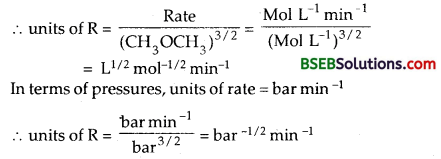Question 5.
Mention the factors^ that affect the rate of a chemical reaction.
There are a number of factors which influence the rate of:
The important factors are –

1. Nature of the reacting species.
2. Concentration of the reacting species.
3. Temperature at which a reaction proceeds.
4. Surface area of the reactants.
6. Presence of a catalyst.

Question 6.
A reaction is second order with respect to a reactant. How is the rate of the reaction affected if the concentration of the reactant is (i) doubled (ii) reduced to half?
Rate = k [A]2 = ka2
if [A] = 2a, rate = k[2a]2 = 4ka2 = 4 times
if [A] = $$\frac { 1 }{ 2 }$$a; rate = k($$\frac { 1 }{ 2 }$$)² = $$\frac { 1 }{ 4 }$$ka2 = $$\frac { 1 }{ 4 }$$th
Thus the rate of a II order reaction becomes 4 times when the concentration of the reactant is doubled and it becomes $$\frac { 1 }{ 4 }$$th when the concentration of reactant is halved.Question 7.
What is the effect of temperature on the rate constant of a reaction? How can this temperature effect on rate constant be represented quantitatively?
The rate constant of a reaction increases with increase in temperature and becomes nearly double for every 10° rise of temperature. The effect can be represented quantitatively by Arrhenius equation.
k = Ae-Ea/RT
where A = constant called frequency factor. It is related to number of binary molecular collisions per second per litre.
Ea = Energy of activation
T = Absolute temperature
N = The integrated form
R = Gas constant
log $$\frac{\mathrm{k}_{2}}{\mathrm{k}_{\mathrm{r}}}$$ = $$\frac{\mathrm{E}_{\mathrm{a}}}{2.303 \mathrm{R}}$$[ $$\left[\frac{T_{2}-T_{1}}{T_{1} T_{2}}\right]$$ ]
where k1 and k2 are the rate constants for the reaction between two different temperatures T1 and T2 respectively.

Question 8.
In a pseudo first order hydrolysis of ester in water the following results were obtained –(i) Calculate the average rate of the reaction between the time interval 30 to 60 seconds.
(ii) Calculate the pseudo first order rate constant for the hydrolysis of ester.
Average rate during the interval 30 – 60 sec.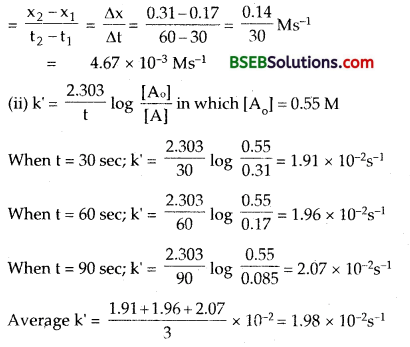Question 9.
A reaction is first order in A and second order in B.
(i) Write the differential rate equation.
(ii) How is the rate affected on increasing the concentration of B three times?
(iii) How is the rate affected when the concentration of both A and B are doubled?
(i) $$\frac { dx }{ dt }$$ = k[A][B]2

(ii) rate = kab2. If [B] is tripled.
Rate = ka.(3b)2 = 9 kab2; rate increases 9 times.

(iii) If both [A] and [B] are doubled
then Rate = k (2a) (2b)2 = 8 kab2 = 8 times.
Rate of the reaction increases 8 times.

Question 10.
In a reaction between A and B, the initial rate of the reaction (r0) was measured for different initial concentrations of A and B as given below: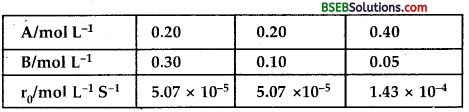What is the order of the reaction with respect to A and B?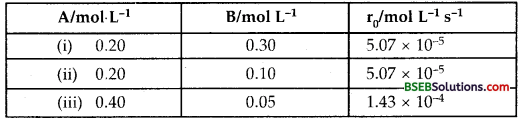Suppose order with respect to A is α and order w.r.t. B is β Then the rate law will be
Rate = k [A]α [B]β
(Rate)expt (i) = 5.07 × 10-5 = k [0.20]α [0.20]β …(i)
(Rate)expt (ii) = 5.07 × 10-5 = k [0.20]α [0.10]β …(ii)
(Rate)expt (iii) = 1.43 × 10-4 = k [0.40]α [0.05]β …(iii)
From (i) and (ii)Question 11.
The following results have been obtained during the kinetic studies of the reaction 2A + B → C + D.Determine the rate law and the rate constant for the reaction.
From Experiment I and IV, it may be noted that [B] is same, but [A] has been made 4 times.
The rate of the reaction also has become four times
∴ Rate w.r.t. A
Rate α [A] … (1)
From experiments II and III, it may be noted that [A] is kept same, and [B] has been doubled, the rate of the reaction has become 4 times. This mean w.r.t. B,
Rate α [B]² … (2)
Combining (1) and (2), we get the rate law of the reaction
2A + B → C + D
Rate Law is Rate = k [A] [B]2
Over – all order of the reaction = 1 + 2 = 3
To calculate rate constant
k = $$\frac{\text { Rate }}{[\mathrm{A}][\mathrm{B}]^{2}}$$ = $$\frac{6.0 \times 10^{-3}}{(0.1)(0.1)^{2}}$$ = 6.0
hence rate constant k = 6.0 M-2 m-1Question 12.
The reaction between A and B is first order with respect to A and zero order with respect to B. Fill in the blanks in the following table:The reaction between A and B is first order with respect to A and zero order with respect to B.
∴ rate of the reaction w.r.t. A = k [A]
rate of the reaction w.r.t.B = k[B]0
∴ overall rate of the reaction = k [A] [B]0
It is of 1st (1 + 0) order.
From I Experiment,
∴ k = $$\frac{\text { Rate }}{[\mathrm{A}][\mathrm{B}]^{\circ}}$$ = $$\frac{2.0 \times 10^{-2}}{[0.1]}$$
= 2.o x 10-1
Now, in Experiment II, the cone, of A will beThe given artefact is 1845 years old.

Question 15.
The experimental data for the decomposition of N2O5 [2N2O5 → 4NO2 + O2] in gas phase at 318 K are given below.(i) Plot [N2O5] against t.
(ii) Find the half-life period for the reaction.
(iii) Draw a graph between log [N2O5] and t.
(iv) What is the rate law?
(v) Calculate the rate constant.
(vi) Calculate the half-life period from k and compare it with (ii).
(a) Plot [N2O5] against t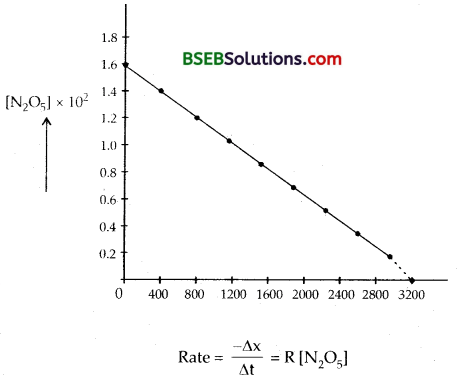The different values of k calculated are 4.96 × 10-4 s-1,
4.82 × 10-4 s-1, 5.0 × 10-4 s-1, 5.44 × 10-4 s-1, 5.44 × 10-4 s-1,
5.47 × 10-4 s-1, 5.91 × 10-4 s-1, 5.81 × 10-4 s-1 and 5.71 × 10-4 s-1.
Since the value of k is almost constant, the rate equation is
γ = k[N2O5]
The mean value of rate constant is 5.2873 × 10-4 s-1 order of the reaction = 1.
Half life period t1/2 = $$\frac{0.693}{\mathrm{k}}$$ = $$\frac{0.693}{5.2873 \times 10^{-4}}$$ = 1310 s.Question 16.
The rate constant for a first order reaction is 60 s-1. How much time will it take to reduce the initial concentration of the reactant to its 1/16 th value?
It is a first order reaction
k = 60 s-1
Let the initial cone, of the reactant = a M L-1
Let t be the time (in seconds) during which its initial concentration reduces to 1/16 of the value.
∴ conc. after time t = $$\frac { a }{ 16 }$$
Putting the values in the 1st order square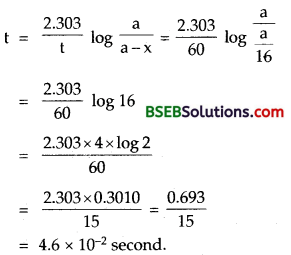Hence after 4.6 × 10-2 second, the initial concentration of the reactant reduces to 1/16.

Question 17.
During nuclear explosion, one of the product is 90Sr with half life of 28.1 years. If 1 pg of 90Sr was absorbed in the bones of a newly born baby instead of calcium, how much of it will remain after 10 years and 60 years if it is not lost metabolically.
Half life period of 90Sr = 28.1 years
decay constant (k) = 0.693/28.1
Amount of 90Sr absorbed in the bones of the newly born baby = 1 μg = 10-6gm.
After time t = 10 years, the amount of radioactive 90Sr = N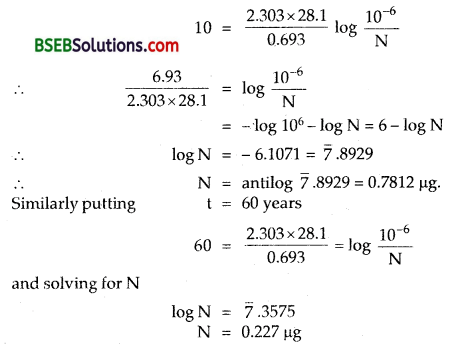∴ Amount of 90Sr left after 10 years, 60 years respectively is 0.7812

Question 18.
For a first order reaction show that the time required for 99% completion is twice the time requiered for the competion of 90% of reaction.Question 19.
A first order reaction takes 40 min for 30%decomposition. Calculation tt/2.Question 20.
For the composition of azoisopropane to hexane and nitrogen at 543 k, the following data are obtained.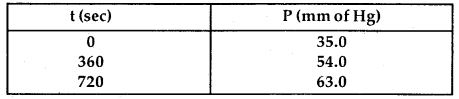Calculate the rate constant.
Azoisopropane decompose according to the equation.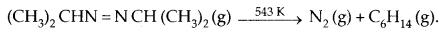The decomposition is found out to be of the first order.
Initial pressure P0 = 35.0 mm of Hg.
Decrease of pressure of azoisopropane after time t = P = p
∴ increase in pr. of N2 = p
and increase in pr. of hexane = p.
Total pressure of the mixture = pt = PA + PN2 + Pc16H14
pt = (P0 – P) + P + P = p0 + P
P = Pt – P0
p = Pt – P0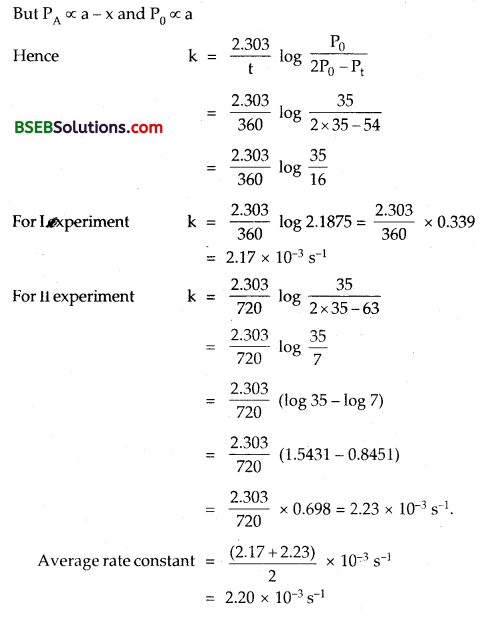Question 21.
The following data were obtained during the first order thermal decomposition of SO2Cl2 at constant volume.
SO2Cl2(g) → SO2 (g) + Cl2(g)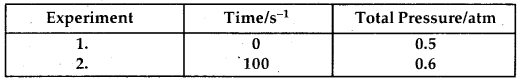Calculate the rate of the reaction when total pressure is 0.65 atm.
1. As in question 4.20= 2.23 × 10-3 s-1
Hence rate constant of the 1st order reaction = 2.23 × lO-3 s-1.

2. To calculate rate of the reaction when total pressure is 0.65 atm.
$$\frac { dx }{ dt }$$ = k(a – x) = (2P0 – Pt) × k
= (1.00 – .65) × 2.23 × lO-3
= 0.35 × 2.23 × lO-3
rate of reaction = 7.8 × 10-4 atm s-1

Question 22.
The rate constant for the decomposition of N2O5 at vsrious temparature is given below: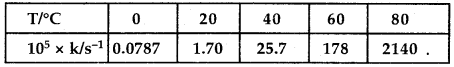Draw a graph between k and 1/T and calculate the value of A and Ea. Predict the rate constant at 30°C and 50°
From the given data, log k is calculated for each value of k.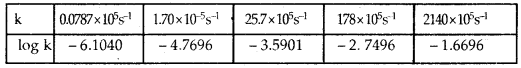On plotting log k versus $$\frac { 1 }{ T }$$, the slope of the line is found to be – 5300. [Students are advised to plot the actual graph on graph paper]
∵ Slope = $$-\frac{E_{a}}{2.303 R}$$
∴ Ea = – 2.303 × 8.314 (-5300)
= 101479.85 JK-1 mol-1
Ea = 101.5 KJ k-1 mol-1

(ii) To calculate the rate constant at 30°C = 303 K.
If T1 = 273 K; k1 = 0.0787 × 10-5
T2 = 303 K K2 = ?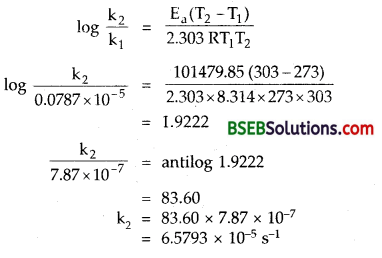Similarly the rate constant at 323 K is 8.14 x 10-4 s-1.

Question 23.
The rate constant for the decomposition of hydrocarbons is 2.418 × 10-5 s-1 at 546 K. If the energy of activation is 179.9 kJ/mol, what will be the value of pre-exponential factor?
Ea = 179.9 = 179900 J
k = 2.418 × 10-5
and T = 546 K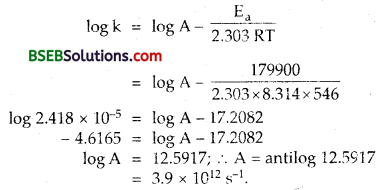Question 24.
Consider a certain reaction A → Products with k = 2.0 × 10-2 s-1. Calculate the concentration of A remaining after 100 s if the initial concentration of A is 1.0 mol L-1.
The reaction A → Products is a first order reaction ask = 2.0 × 10-2 second L-1.
[The value of k in second-1 indicates it is 1st order reaction]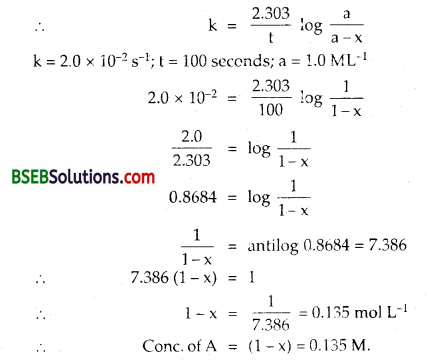Question 25.
Sucrose decomposes in acid solution into glucose and fructose according to the first order rate law with T1/2 = 3.00 hours. What fraction of sample of sucrose remains after 8 hours?
Since it follows rate law of first order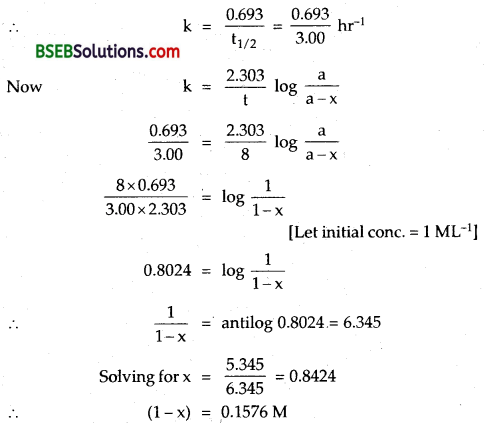fraction of sample of sucrose remaining = 0.1576 M

Question 26.
The decomposition of hydrocarbon follows the equation k = 4.5 × 1011 s-1 e28000k/t Calculate Ea.
log k = log A – $$-\frac{E_{a}}{2.303 R}$$ … (i)
The decomposition of the hydrocarbon follows the equation.
k = 4.5 × 1011 s-1 e28000k/t
Applying logs
log k = log 4.5 × 1011 – $$\frac{28000}{\mathrm{~T}}$$
log10 k = log10 4.5 × 1011 – $$\frac{28000}{2.303 T}$$ K … (ii)
Comparing it with equation no. (i)
$$-\frac{E_{a}}{2.303 R}$$ = $$\frac{28000}{2.303 T}$$
∴ Ea – 28,000 x 8.314 J
= 28 x 8.314 kJ mol-1
Ea = 232.79 kJ mol-1.

Question 27.
The rate constant for the first order decomposition of H2O2</su-2 is given by the following equation:
logk = 14.34 – 1.25 x 104 $$\frac { K }{ T }$$
Calculate Ea for the reaction and at what temperature will its half-period be 256 minutes?
(i) log k = log A – $$-\frac{E_{a}}{2.303 R}$$
The given equation is
log k = 14.34 – $$\frac{1.25 \times 10^{4}}{T}$$ K
Comparing the two equations
$$-\frac{E_{a}}{2.303 R}$$ = 1.25 x 104
or Ea = 1.25 x 104 x 2.303 x 8.314 J mol-1
= 239.34 k] mol-1

(ii) t1/2 =256 min = 256 x 60 seconds.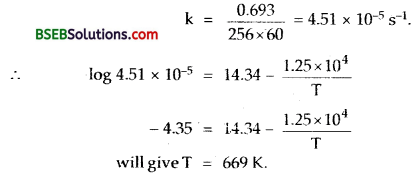Question 28.
The decomposition of A into product has value of k as 4.5 × 10-3 s-1 at 10°C and energy 60kJ mol-1. At what temparature would k be 1.5 × 104 s-1 ?Question 29.
The time required for 10% completion of a first order reaction at 298 k is equal to that required for its 25% completion at 308 k. if the value of the A is 4 × 1010 s-1, calculate k at 318 k and EaPutting A = 4 x 1010 s-1; R = 8.314 x 10-3 x 10-3 JK-1 mol-1,
Ea kJ mol-1, T = 318 k in the above equation,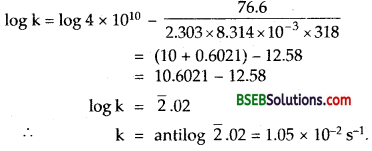Question 30.
The rate of a reaction quadruples when the temperature changes from 293 K to 313 K. Calculate the energy of activation of the reaction assuming that it does not change with temperature.
T1 = 293;
T2 = 313 K
T2 – T1 = 20°
k2 = 4k1### Bihar Board Class 12 Chemistry Chemical Kinetics Additional Important Questions and Answers

Question 1.
After 5 half-life periods for a first order reaction what fraction of reactant remains?
$$\frac { 1 }{ 2 }$$.

Question 2.
A reaction is found to be zero order. Will its molecularity be also zero?
No. Molecularity of reaction cannot be zero.

Question 3.
Is there any reaction for which the rate of a reaction does not decrease with time?
For a zero order reaction, the reaction rate does not decrease with time because it is independent of the concentration of the reactants.Question 4.
Can activation energy for reactants be zero?
In the Arrhenius equation, k = A g-Ea/RT if Ea = 0 then k = A, i.e. every collision between molecules leads to chemical reaction. This is not true. Thus Ea cannot be zero.

Question 5.
What value of K is predicted for the rate constant by Arrhenius equation if T → ∞? Is this value physically reasonable?
From the Arrhenius equation k = A e-Ea/ RT if T → ∞; k → A so that Ea = 0. This is not feasible.

Question 6.
Why AG is postive for some of the photochemical reactions?
This is because a part of the light energy absorbed by the reactants gets converted into free energy.

Question 7.
For a reaction A + H2O → B; rate α [A]. What is its (i) molecularity (ii) order of the reaction?
It is a Pseudo unimolecular reaction with order = 1.

Question 8.
What is the main difference between a photosenstitizer and a catalyst?
A catalyst only alters (changes) the speed of the reaction, while a photosensititizer only initiates the reaction.

Question 9.
How is the rate constant related to concentration of the reactants?
Rate constant does not depend upon the concentration of the reactants.

Question 10.
The rate Law for the decomposition of N2O5 is
Rate = k [N2O2] (A.LS.E. 2002)
What is the significant ace of k in this equation?
If [N2O5] = 1; then Rate = k.
i.e. k is equal to the rate of the reaction when concentration of N2O5 is unity, i.e., 1 mol L-1.

Question 11.
Write expression for half-life in case of a reaction between hydrogen and chlorine to form hydrochloric acid gas. (P.S.B. 2005)
Given reaction is a zero order reaction. Hence 1/2 = [A]0/2k.Question 12.
For the assumed reaction X2 + 3Y2 → 2XY3, write the rate equation in terms of rate of disappearance of Y2. (H.S.B. 2005)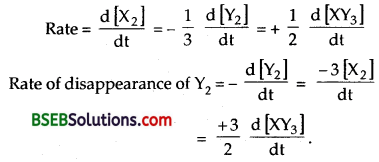Question 13.
The reaction A + B → C has zero order. What is the rate equation? (A,I,S,B. 2005)
Rate = k [A]° [B]° = k.

Question 14.
Express the relation between half life period of a reactant with initial concentration for a reaction of nth order. (A,I,S,B. 2005)
t1/2 α $$\frac{1}{[\mathrm{~A}]^{\mathrm{n}-1}}$$.

Question 15.
How does the value of rate constant vary with reactant concentration? (D.S.B. 2005)
For reaction of nth order $$\frac { dx }{ dt }$$ = Rate = k [conc.]nQuestion 16.
If half-life period of a reaction is inversely proportinal to initial concentration of a reactant, what is the order of the reaction? (C.B.S.E. Sample Paper 2003)
Two, this is because for 2nd order reactions t1/2 1/ka.

Question 17.
Give one example of a first order reaction. (A.I.S.B. 1998)
N2 O5 → N2 O4 + $$\frac { 1 }{ 2 }$$ O2

Question 18.
For a reaction A → B, the rate of the reaction can be denoted by-dA/dt-ar+dB/dt State the significance of plus and minus signs in this case. (H.S.B.2001)
Minus sign indicates the decrease in the concentration of the reactant while plus sign indicates an increase in the cone, of the product with time.

Question 19.
Why rate of reaction does not remain constant throughout? (P.S.B.2000)
Because it depends upon concentration of the reactions which keeps on decreasing with time.

Question 20.
What is the relationship between rate constant and activation energy of a reaction? (H.S.B. 1999, H.P.S.B. 2004)
k = A e-Ea/RT is Arrhenius equation.Question 21.
Why hydrolysis of ethyl acetate with NaOH is a second order reaction while with HCl it is a 1st order reaction?
Rate of hydrolysis of ethyl acetate with NaOH depends upon cone, of both while that with HCl, it depends upon only the cone, of ethyl acetate.

Question 22.
Define specific reaction rate or rate constant. (P.S.B.2000)
It is the rate of a reaction when the molar concentration of each of the reactant is unity.

Question 23.
What is the rate determineg step of a reaction?
The slowest step of a multi-step reaction is called its rate-determining step.

Question 24.
Give an example of a reaction having fractional order.
Decomposition of acetaldehyde (order is 1.5)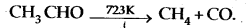Question 25.
A substance with initial concentration ‘a’ follows zero order kinetics. In how much time will the reaction go to completion? (D.S.B. 2005)
$$\frac { dx }{ dt }$$ = k or dx = k dt ∴ x = kt + I
when t = 0; x = 0 ∴ I = 0.
Hence x = kt or t = x / k, For completion x = a
∴ t = a/k.

Question 26.
In some cases, it is found that a large no. of colliding molecules have energy more than threshold energy, yet the reaction is slow, why? (A.I.S.B. 2005)
The colliding molecules may not have proper orientation at the time of collision.

Question 27.
Give an example of a pseudo first order reaction. (A.I.S.B. 2004, D.S.B. 2004)
Acid catalysed hydrolysis of ethyl acetate.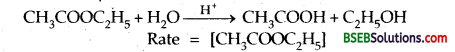Question 28.
What is meant by an elementary reaction? (A.I.S.B. 2004)
A reaction which takes place in one step is called an elementary reaction.

Question 29.
How is half-life period related to initial concentration of a second order reaction? (D.S.B. 2004 C)
t1/2 α a1-n. Forn = 2, t1/2 α a-1, i.e. t1/2 α $$\frac { 1 }{ a }$$. Thus half life period of a second order reaction is inversely proportional to the initial concentration of the reactant.Question 30.
Give one example of a reaction where order and molecularity are equal. (C.B.S.E. Sample Paper 1997)
Order and molecularity are same in a single step reaction.
example: 2HI(g) → H2 (g) + I2 (g)

Question 31.
State any one condition under which a bimolecular reaction may be kinetically of first order. (D.S.B. 2002)
A bimolecular reaction may become kinetically of the first order if one of the reactants is present in excess.

Question 32.
Rate of a reaction is given by the equation: Rate = k [A]² [B]. What are the units for the rate and rate constant for this reaction? (D.S.B. 2001)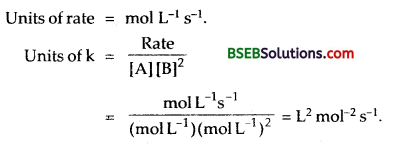Question 33.
How is the rate constant of a reaction related to the concentration of the reactants?
Rate constant does not depend upon the concentration of the reactants.

Question 34.
The rate law for the reaction: Ester + H+ → Acid + alcohol is dx/dt = k [Ester] [H+]°. What would be the effect on the rate if (i) concentration of the ester is doubled, (ii) concentration of H+ is doubled? (P.S.B.2002)

• The rate of the reaction will be doubled.
• No effect on rate.

Question 35.
The form of the rate law for a reaction is expressed as: rate = k [Cl2] [NO]². Find out the orders of the reaction with respect to Cl2 and with respect to NO and also the overall order of the reaction. (A.I.S.B. 1994)
Order w.r.t. Cl2 = 1; Order w.r.t. NO = 2
Over-all order = 1 + 2 = 3.Question 1.
Write the expressions for rates of reactions in terms of concentration of reactants and products for the following reactions:
(i) 2 N2O5 $$\rightleftharpoons$$ 2N2O4 + O2
(ii) 2NO2 + F2 $$\rightleftharpoons$$ 2NO2F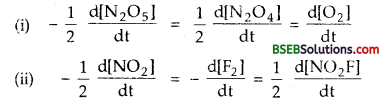Question 2.
Assuming that energy of activation for most of the reactions is 52 kJ, what conclusion you draw about the effect of temperature on the rate of a reaction [Based on Arrhenius equation]? (P.S.B. 2005)
Putting T1 = 300 K, T2 = 310 K, Ea = 52,000 J mol-1 in the Arrhenius equation,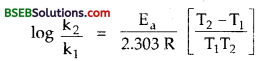we get k2 = 2k1 This shows that for 10° rise of temperature, the rate of the reaction is doubled.

Question 3.
The mechanisms of the photosynthesis of HCl and HBr are similar, yet the quantum efficiency of the former is high while that of latter is very low. Why?
This is because the first stage of the secondary process in the first case, viz., Cl + H2 → HCl + H is exothermic while in the 2nd case Hr + H2 → HBr + H, it is endothermic.

Question 4.
A first order reaction takes 69.3 minutes for 50% completion. Set up an equation for determining the time needed for 80% completion of this reaction (Calculation of result is not required.) (D.S.B. 2005)
t1/2 = 69.3 min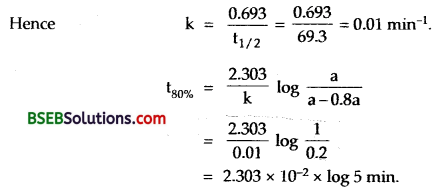Question 5.
For the gaseous reaction
2NO2 (g) → 2NO (g) + O2 (g)
(i) Write the expression for rate of the reaction.
(ii) If the rate of decrease of concentration of NO2 is 6.0 x 10-12 s-1, calculate the corresponding rates in terms of increase in NO and O2 concentrations.
(i) Rate = k[NO2
(ii) Rate of increase of NO. concentration = 6.0 x 10-12 s-1
Rate of increase in O2 concentration = 3.0 x 10-12 s-1.

Question 6.
(a) Draw a schematic graph showing how the rate of a first order reaction changes with change in concentration of a reactant.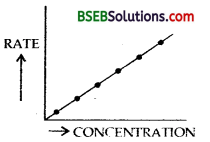Rate of 1st order reaction increase with -the increase in concentration as shown in the RATE schematic graph.Question 7.
What type of plot do you expect for the rate versus time for a reaction of zero order?
A straight line parallel to time axis because rate is independent of concentration and hence time.Question 8.
Following reaction takes place in one step :
2NO (g) + O2 (g) $$\rightleftharpoons$$ 2NO2 (g)
How will the rate of the above reaction change if the volume of the reaction vessel is diminished to one-third of the original volume? Will there be any change in the order of the reaction with reduced volume? (A.I.S.B. 2004)
Rate = K[NO]²[O2]
[NO] = M, [O2] = $$\frac { a }{ V }$$ M; Let Moles of NO = a and moles of O2 = b and Vol. of the vessel = V litres.∴ r2/r1 or r2 = 27r1; rate becomes 27 times.
There is no effect on the order of the reaction.

Question 9.
The rate expressions of some reactions are given below:
(a) 2NO2 + F2 → 2NO2F; Rate = k [NO2] [F2]
(b) NO2 + CO → CO2 + NO; Rate = k [NO2]².
Propose the probable mechanism of each of the above reactions.
(a)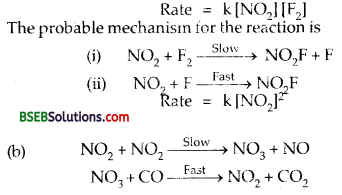Question 19.
What is a photochemcial reaction? Define quantum efficiency of a photochemical reaction.
Photochemical reaction is one which can proceed in the presence of light (or some other radiation). Quantum efficiency (Φ) is expressed as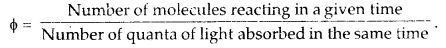Question 1.
(a) What is the difference between ORDER and MOLECULARITY of a reaction?
(b) RATE of a reaction and RATE CONSTANT of a reaction?
(c) What are the factors which influence the rate of reaction.
Discuss them in detail.
(a) Difference between ORDER & MOLECULARITY

Molecularity:

• It is the number of reacting species undergoing simul – taneous collision in the reaction.
• It is a theoretical concept.
• It can have integral values only.
• It cannot be zero.
• It does not tell us anything about the mechanism of the reaction.

Order:

• It is sum of the powers of the concentration terms in the rate law expression.
• It is determined experi-mentally.
• It can have fractional values also.
• It can be zero.
• It tells us about the slowest step in the mechanism and hence gives some clue about mechanism of the reaction:

(b) Difference between RATE of a reaction and RATE CONSTANT.

Rate of Reaction:

• It is the speed at which the reactants are converted into products at any moment of time.
• It depends upon the concentration of reactant species at that moment of time.
• It decreases with the progress of reaction generally.
• It has same units for all reactions ML-1S-1.

Rate constant of reaction

• It is a constant of proportionality in the rate law expression.
• It refers to the rate of the reaction at the specific point when concentration of every reacting species is unity.
• It is constant and does not depend on the progress of the reaction.
• It has different units for different reactions.

(c) (1) Nature of the reacting species – Consider the following two reactions:
2NO (g) + O2 (g) → 2NO2 (g) …fast
2CO (g) + O2 (g) → 2CO2 (g) …slow
These reactions appear to be similar but the first is fast, while the second is slow. This is because different amounts of energies are required for breaking of different bonds and different amounts of energies are released in the formation of different bonds.

2. Concentration of the reactants – Greater are the concentrations of the reactants, faster is the reaction. Conversely, if the concentrations of the reactants decrease, the rate of the reaction also decreases. A match stick which burns usually in air (02:21% by volume) bums with a flash in a jar containing 02 gas (volume of 07:100%).

3. Temperature – The rate of a reaction increases with the increase in temperature. In most of the reactions the rate of the reaction gets doubled for 10°C rise in temperature irrespective of the fact whether the reaction is exothermic or endothermic. In some cases reactions do not take place at room temperature but proceed only on heating.

4. Surface area of the reactants – For a reaction involving a solid reactant or catalyst, the smaller is the particle size, i.e., greater is the surface area, the faster is the reaction. Wood shavings bum rapidly in air than a log of wood, because the surface area of wood shavings is much higher.

5. Pressure of a catalyst – A catalyst generally increases the speed of the reaction without itself being consumed in the reaction. In case of reversible reactions a catalyst helps attain chemical equilibrium quickly without disturbing the state of equilibrium. It speeds up the reaction both in the forward as well as backward direction so that equilibrium is attained earlier.

6. Presence of light – Some reactions do not take place in the dark, but take place in the presence of light.
Exaple: H2 + Cl2 → 2HCl
Such reactions are called PHOTO-CHEMICAL REACTIONS.Question 2.
(a) For the reaction
4 NH3 (g) + 5O2 (g) → 4NO (g) + 6H2O (g)
if the rate expression in terms of disapperance of NH3 is – ∆ (NH3)/∆T, write the rate expression in terms of concentration of O2 and H2O,

(b) In the reaction A → B, the value of the rate constant was found to be 1.0 x 10-2 mol-1 L S-1. What is the order of the reaction? How will the catalyst affect the values of the rate constant? (C.B.S.E. Sample Paper 1997)

(c) Identify the order if in a reaction, the rate constant has the units L Mol-1 S-1.

(d) A first order reaction is found to have a rate constant k = 7.39 x 10-5 sec-1 Find the half life period of a reaction.

(e) Explain the process of inversion of cane-sugar.
(a) Rate = – $$\frac { 4 }{ 5 }$$$$\frac{\Delta\left[\mathrm{O}_{2}\right]}{\Delta \mathrm{t}}$$ = $$\frac { 4 }{ 6 }$$.$$\frac{\Delta \mathrm{H}_{2} \mathrm{O}}{\Delta \mathrm{t}}$$